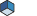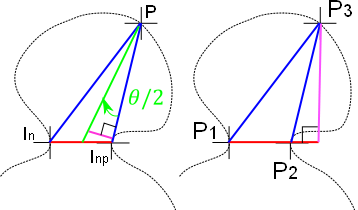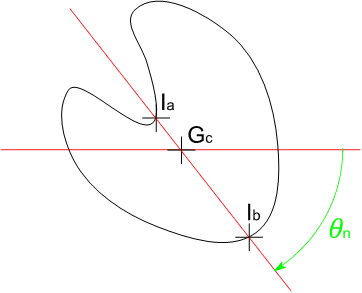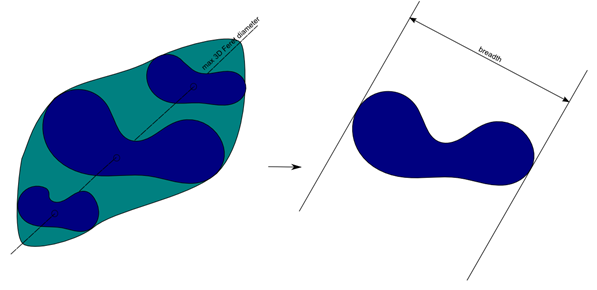# SoDataMeasurePredefined Class Reference [Measures]class that define the list of predefined measure that can be used on image data input. More...

#include <ImageViz/Nodes/Measures/SoDataMeasurePredefined.h>

Inheritance diagram for SoDataMeasurePredefined:List of all members.

## Public Types

enum  PredefinedMeasure {
UNDEFINED = 0,
ANISOTROPY,
AREA_2D,
AREA_3D,
VOLUME_FRACTION,
BARYCENTERX,
BARYCENTERY,
BARYCENTERZ,
MOMENT2X,
MOMENT2Y,
MOMENT2Z,
MOMENTXY,
MOMENTXZ,
MOMENTYZ,
BORDER_VOXEL_COUNT,
BOUNDING_BOX_DX,
BOUNDING_BOX_DY,
BOUNDING_BOX_DZ,
BOUNDING_BOX_OX,
BOUNDING_BOX_OY,
BOUNDING_BOX_OZ,
SMOOTH_CIRCLE_DIFFERENCE_2D,
CONVEX_AREA_2D,
CONVEX_PERIMETER_2D,
CROFTON_PERIMETER_2D,
NEIGHBOR_DISTANCE_2D,
EIGEN_VALUE1,
EIGEN_VALUE2,
EIGEN_VALUE3_3D,
EIGEN_VECTOR1X,
EIGEN_VECTOR1Y,
EIGEN_VECTOR1Z_3D,
EIGEN_VECTOR2X,
EIGEN_VECTOR2Y,
EIGEN_VECTOR2Z_3D,
EIGEN_VECTOR3X_3D,
EIGEN_VECTOR3Y_3D,
EIGEN_VECTOR3Z_3D,
ENCLOSING_ELLIPSE_DIFFERENCE_2D,
ELONGATION,
ENCLOSING_CIRCLE_DIFFERENCE_2D,
EQUIVALENT_DIAMETER,
EULER_2D,
EULER_3D,
ECCENTRICITY_2D,
EXTENT_MAX1,
EXTENT_MAX2,
EXTENT_MAX3_3D,
EXTENT_MIN1,
EXTENT_MIN2,
EXTENT_MIN3_3D,
FERET_DIAMETER_2D,
FERET_INPUTX_2D,
FERET_INPUTY_2D,
FERET_OUTPUTX_2D,
FERET_OUTPUTY_2D,
FERET_DIAMETER_RATIO_2D,
FERET_DIAMETER_RATIO_3D,
FIRST_POINTX,
FIRST_POINTY,
FIRST_POINTZ,
FLATNESS_3D,
GRAY_BARYCENTERX,
GRAY_BARYCENTERY,
GRAY_BARYCENTERZ,
HISTOGRAM_KURTOSIS,
HISTOGRAM_MEAN,
HISTOGRAM_PEAK,
HISTOGRAM_QUANTILE10,
HISTOGRAM_QUANTILE25,
HISTOGRAM_QUANTILE50,
HISTOGRAM_QUANTILE75,
HISTOGRAM_QUANTILE90,
HISTOGRAM_QUANTILER1,
HISTOGRAM_QUANTILER2,
HISTOGRAM_QUANTILER3,
HISTOGRAM_QUANTILER4,
HISTOGRAM_QUANTILER5,
HISTOGRAM_QUANTILER6,
HISTOGRAM_SKEWNESS,
HISTOGRAM_STDDEV,
HISTOGRAM_VARIANCE,
INSIDE_LENGTH_2D,
LENGTH_2D,
LENGTH_3D,
LENGTH_ORIENTATION_PHI_3D,
LENGTH_ORIENTATION_THETA_3D,
LENGTH_ORIENTATION_2D,
INTENSITY_MAJORITY,
INTENSITY_MAXIMUM,
INTENSITY_MEAN,
INTENSITY_MEDIAN,
INTENSITY_MINIMUM,
HOLES_COUNT_2D,
ORIENTATION_2D,
ORIENTATION2_PHI_3D,
ORIENTATION2_THETA_3D,
ORIENTATION1_PHI_3D,
ORIENTATION1_THETA_3D,
BOUNDARY_VOXEL_COUNT,
RUGOSITY_2D,
POLYGONE_AREA_2D,
RECTANGLE_CENTERX_2D,
RECTANGLE_CENTERY_2D,
RECTANGLE_LENGTH_2D,
RECTANGLE_ORIENTATION_2D,
RECTANGLE_WIDTH_2D,
SEGMENT_HOLES_COUNT_2D,
POLYGONE_PERIMETER_2D,
SHAPE_FACTOR_AP_2D,
SHAPE_FACTOR_VA_3D,
INTENSITY_STANDARD_DEVIATION,
SYMMETRY_2D,
INTENSITY_INTEGRAL,
SQUARE_INTENSITY_INTEGRAL,
VOLUME_3D,
VOXEL_FACE_AREA_3D,
WIDTH_2D,
WIDTH_3D,
WIDTH_ORIENTATION_PHI_3D,
WIDTH_ORIENTATION_THETA_3D,
WIDTH_ORIENTATION_2D,
THICKNESS_3D,
THICKNESS_ORIENTATION_PHI_3D,
THICKNESS_ORIENTATION_THETA_3D,
GRAY_ANISOTROPY,
GRAY_EIGEN_VALUE1,
GRAY_EIGEN_VALUE2,
GRAY_EIGEN_VALUE3_3D,
GRAY_EIGEN_VECTOR1X,
GRAY_EIGEN_VECTOR1Y,
GRAY_EIGEN_VECTOR1Z_3D,
GRAY_EIGEN_VECTOR2X,
GRAY_EIGEN_VECTOR2Y,
GRAY_EIGEN_VECTOR2Z_3D,
GRAY_EIGEN_VECTOR3X_3D,
GRAY_EIGEN_VECTOR3Y_3D,
GRAY_EIGEN_VECTOR3Z_3D,
GRAY_ELONGATION,
GRAY_EXTENT_MAX1,
GRAY_EXTENT_MAX2,
GRAY_EXTENT_MAX3_3D,
GRAY_EXTENT_MIN1,
GRAY_EXTENT_MIN2,
GRAY_EXTENT_MIN3_3D,
GRAY_FLATNESS_3D,
GRAY_MOMENT2X,
GRAY_MOMENT2Y,
GRAY_MOMENT2Z,
GRAY_MOMENTXY,
GRAY_MOMENTXZ,
GRAY_MOMENTYZ,
INTENSITY_COUNT,
NEIGHBOR_COUNT,
LENGTH_INPUT_X_3D,
LENGTH_INPUT_Y_3D,
LENGTH_INPUT_Z_3D,
LENGTH_OUTPUT_X_3D,
LENGTH_OUTPUT_Y_3D,
LENGTH_OUTPUT_Z_3D,
WIDTH_INPUT_X_3D,
WIDTH_INPUT_Y_3D,
WIDTH_INPUT_Z_3D,
WIDTH_OUTPUT_X_3D,
WIDTH_OUTPUT_Y_3D,
WIDTH_OUTPUT_Z_3D,
THICKNESS_INPUT_X_3D,
THICKNESS_INPUT_Y_3D,
THICKNESS_INPUT_Z_3D,
THICKNESS_OUTPUT_X_3D,
THICKNESS_OUTPUT_Y_3D,
THICKNESS_OUTPUT_Z_3D,
VOXEL_COUNT,
INSIDE_CENTER_X_2D,
INSIDE_CENTER_Y_2D
}

## Public Member Functions

virtual SoType getTypeId () const
SoDataMeasurePredefined ()
SoDataMeasurePredefined (PredefinedMeasure measure)
virtual const SbStringgetMeasureName () const
virtual UnitDimension getUnitDimension () const
virtual ResultFormat getFormat () const

## Static Public Member Functions

static SoType getClassTypeId ()
static const SbStringgetName (PredefinedMeasure measureEnum)

## Public Attributes

SoSFEnum predefinedMeasure

## Detailed Descriptionclass that define the list of predefined measure that can be used on image data input.

For an introduction see section Measure Node .

See related examples:

## Member Enumeration Documentation

Enumeration of all pre defined measure that can be used.

Enumerator:
 UNDEFINED Default value is undefined. It will be ignored by analysis request ANISOTROPY 1 minus the ratio of the smallest to the largest eigenvalue of the covariance matrix. Measures a region's deviation from a spherical shape.AREA_2D Area of the object. See also the documentation of the SoAreaQuantification2d engine. AREA_3D Area of the object boundary. See also the documentation of the 3D interpretation of the SoAreaQuantification2d engine. VOLUME_FRACTION Ratio between the label area and the image area in 2D:Ratio between the label volume and the image volume in 3D:. BARYCENTERX X coordinate of the center of gravity. BARYCENTERY Y coordinate of the center of gravity. BARYCENTERZ Z coordinate of the center of gravity. MOMENT2X Centered moment term (XX) of the covariance matrix. MOMENT2Y Centered moment term (YY) of the covariance matrix. MOMENT2Z Centered moment term (ZZ) of the covariance matrix. MOMENTXY Centered moment term (XY) of the covariance matrix. MOMENTXZ Centered moment term (XZ) of the covariance matrix. MOMENTYZ Centered moment term (YZ) of the covariance matrix. BORDER_VOXEL_COUNT Some components of the image volume might be intersected by the Bounding Box of the image volume. This value is the number of voxels that are touching this boundary. The component might not be fully within the image. BOUNDING_BOX_DX Width of the Bounding Box. BOUNDING_BOX_DY Height of the Bounding Box. BOUNDING_BOX_DZ Depth of the Bounding Box. BOUNDING_BOX_OX X coordinate of the upper-left corner of the Bounding Box. BOUNDING_BOX_OY Y coordinate of the upper-left corner of the Bounding Box. BOUNDING_BOX_OZ Z coordinate of the upper-left corner of the Bounding Box. SMOOTH_CIRCLE_DIFFERENCE_2D Difference between the object area A and the area of the smoothing circle AS ( Union(A,AS) - Intersection(A,AS) ). The smoothing circle is computed on the polygonal approximation of the object boundary and verifies : the center of the circle is the barycenter of the object the distance between the circle and the approximated polygon minimizes the least squares criteria CONVEX_AREA_2D Area of the convex hull of the polygonal approximation of the object boundary. CONVEX_PERIMETER_2D Perimeter of the convex hull of the polygonal approximation of the object boundary. CROFTON_PERIMETER_2D For each of the 8 intercept measures, the diametral variation is the product of intercept with the distance between interception points. The Crofton perimeter is the average of those 8 diametral variation measures. See Intercept count. NEIGHBOR_DISTANCE_2D Gives the shortest edge to edge distance from the current object to its nearest neighbor. The distance measure is computed from the polygonal approximation of the object boundaries. EIGEN_VALUE1 The largest eigenvalue of the covariance matrix. EIGEN_VALUE2 The medium 3D eigenvalue or smallest 2D eigenvalue of the covariance matrix. EIGEN_VALUE3_3D The smallest 3D eigenvalue of the covariance matrix. This value is only available in 3D. EIGEN_VECTOR1X X coordinate of the largest eigenvector of the covariance matrix. EIGEN_VECTOR1Y Y coordinate of the largest eigen vector of the covariance matrix. EIGEN_VECTOR1Z_3D Z coordinate of the largest eigenvector of the covariance matrix. This value is only available in 3D. EIGEN_VECTOR2X X coordinate of the medium 3D eigenvector or smallest 2D eigenvector of the covariance matrix. EIGEN_VECTOR2Y Y coordinate of the medium 3D eigenvector or smallest 2D eigenvector of covariance matrix. EIGEN_VECTOR2Z_3D Z coordinate of the medium 3D eigenvector or smallest 2D eigenvector of the covariance matrix. This value is only available in 3D. EIGEN_VECTOR3X_3D X coordinate of the 3D smallest eigenvector of the covariance matrix. This value is only available in 3D. EIGEN_VECTOR3Y_3D Y coordinate of the 3D smallest eigenvector of the covariance matrix. This value is only available in 3D. EIGEN_VECTOR3Z_3D Z coordinate of the 3D smallest eigenvector of the covariance matrix. This value is only available in 3D. ENCLOSING_ELLIPSE_DIFFERENCE_2D Difference between the area A of the studied particle and the area of its enclosing ellipse EA ( Union(A,EA) - Intersection(A,EA) ). The enclosing ellipse is computed on the polygonal approximation of the object boundary and verifies: the center of the ellipse is the barycenter of the object the major axis of the ellipse is parallel to the inertia axis of the object the length of the minor and major axis is given by the extent size of the eigenvectors of the covariance matrix. ELONGATION The ratio of the medium and the largest eigenvalue of the covariance matrix. Elongated objects will have small values close to 0.ENCLOSING_CIRCLE_DIFFERENCE_2D Difference between the object area A and the area of the enclosing circle EA ( Union(A,EA) - Intersection(A,EA) ). The enclosing circle is computed on the polygonal approximation of the object boundary. EQUIVALENT_DIAMETER The diameter of the circular particle of same area in 2D:The diameter of the spherical particle of same volume in 3D:. EULER_2D 1 minus the number of holes. See Euler Number section. EULER_3D ECCENTRICITY_2D The eccentricity is defined as:andare the covariance matrix eigenvalues. A disk or a cross has a null eccentricity since. The eccentricity increases with the difference between the eigenvalues, and thus measures the elongation of the object. It also indicates in some cases a privileged direction, corresponding to a large eigenvalue and a small one, i.e.:has a high value, though two orthogonal privileged directions mean two large eigenvalues and a smaller difference. EXTENT_MAX1 The maximal extent of the data in the direction of the largest eigenvector of the covariance matrix. EXTENT_MAX2 The maximal extent of the data in the direction of the medium (second) eigenvector of the covariance matrix. EXTENT_MAX3_3D The maximal extent of the data in the direction of the smallest eigenvector of the covariance matrix. This value is only available in 3D. EXTENT_MIN1 The minimal extent of the data in the direction of the largest eigenvector of the covariance matrix. EXTENT_MIN2 The minimal extent of the data in the direction of the medium (second) eigenvector of the covariance matrix. EXTENT_MIN3_3D The minimal extent of the data in the direction of the smallest eigenvector of the covariance matrix. This value is only available in 3D. FERET_DIAMETER_2D Distribution of the length of Bounding Boxes oriented along the specified directions. See also Feret's Diameter. FERET_INPUTX_2D X coordinate of input point for Feret Distribution. FERET_INPUTY_2D Y coordinate of input point for for Feret Distribution. FERET_OUTPUTX_2D X coordinate of output point for for Feret Distribution. FERET_OUTPUTY_2D Y coordinate of output point for for Feret Distribution. FERET_DIAMETER_RATIO_2D FERET_DIAMETER_RATIO_3D This shape factor is defined as (D/d), where d is the minimum Feret's Diameter and D is the maximum Feret diameter in the orthogonal direction (90 deg from the minimum Feret diameter). Feret measures rely on sampling in a distribution of different directions (31 directions by default). FIRST_POINTX X coordinate of the first point of each object encountered when scanning the input image from top to bottom, and left to right. FIRST_POINTY Y coordinate of the first point of each object encountered when scanning the input image from top to bottom, and left to right. FIRST_POINTZ Z coordinate of the first point of each object encountered when scanning the input image from top to bottom, and left to right. FLATNESS_3D The ratio of the smallest and the medium eigenvalue of the covariance matrix. Flat objects have small values close to 0.See Variance-covariance matrix. This value is only available in 3D. GRAY_BARYCENTERX X coordinate of the center of gravity weighted by the gray level intensities. GRAY_BARYCENTERY Y coordinate of the center of gravity weighted by the gray level intensities. GRAY_BARYCENTERZ Z coordinate of the center of gravity weighted by the gray level intensities. HISTOGRAM_KURTOSIS Kurtosis of the histogram of intensity values within the object. HISTOGRAM_MEAN Mean of the histogram of intensity values within the object. HISTOGRAM_PEAK Peak of the histogram of intensity values within the object. HISTOGRAM_QUANTILE10 10% quantile of the histogram of intensity values within the object. HISTOGRAM_QUANTILE25 25% quantile of the histogram of intensity values within the object. HISTOGRAM_QUANTILE50 50% quantile of the histogram of intensity values within the object. HISTOGRAM_QUANTILE75 75% quantile of the histogram of intensity values within the object. HISTOGRAM_QUANTILE90 90% quantile of the histogram of intensity values within the object. HISTOGRAM_QUANTILER1 Modifiable quantile of the histogram of intensity values within the object (default is 20%). The quantile value can be customized with the SoDataMeasureAttributes::quantilePercents field. HISTOGRAM_QUANTILER2 Modifiable quantile of the histogram of intensity values within the object (default is 30%). The quantile value can be customized with the SoDataMeasureAttributes::quantilePercents field. HISTOGRAM_QUANTILER3 Modifiable quantile of the histogram of intensity values within the object (default is 40%). The quantile value can be customized with the SoDataMeasureAttributes::quantilePercents field. HISTOGRAM_QUANTILER4 Modifiable quantile of the histogram of intensity values within the object (default is 50%). The quantile value can be customized with the SoDataMeasureAttributes::quantilePercents field. HISTOGRAM_QUANTILER5 Modifiable quantile of the histogram of intensity values within the object (default is 60%). The quantile value can be customized with the SoDataMeasureAttributes::quantilePercents field. HISTOGRAM_QUANTILER6 Modifiable quantile of the histogram of intensity values within the object (default is 70%). The quantile value can be customized with the SoDataMeasureAttributes::quantilePercents field. HISTOGRAM_SKEWNESS Skewness of the histogram of intensity values within the object. HISTOGRAM_STDDEV Standard deviation of the histogram of intensity values within the object. HISTOGRAM_VARIANCE Variance of the histogram of intensity values within the object. INSIDE_LENGTH_2D Length of the skeleton of the polygonal approximation of the object boundary. LENGTH_2D Maximum of the Feret Diameters. LENGTH_3D Maximum of the Feret Diameters. LENGTH_ORIENTATION_PHI_3D Angle Phi of the maximum of the Feret's Diameter, in degrees (not radians) [-90,90]. LENGTH_ORIENTATION_THETA_3D Angle Theta of the maximum of the Feret's Diameter, in degrees (not radians) [0,180]. LENGTH_ORIENTATION_2D Angle of the maximum of the Feret Diameters. INTENSITY_MAJORITY The most represented pixel intensity value inside the particle. It uses the nearest value with the average intensity of the particle if several intensities met this criterion. INTENSITY_MAXIMUM Maximum pixel intensity of the particle. INTENSITY_MEAN Average pixel intensity intensity. INTENSITY_MEDIAN INTENSITY_MINIMUM Minimum pixel intensity of the particle. HOLES_COUNT_2D Number of holes of the particle. ORIENTATION_2D Orientation of the particle in degrees (not radians) [-90,+90] computed with the inertia moments. ORIENTATION2_PHI_3D Phi minor orientation of the particle in degrees (not radians) [0,+90], computed with the inertia moments. ORIENTATION2_THETA_3D Theta minor orientation of the particle in degrees (not radians) [-180,+180], computed with the inertia moments. ORIENTATION1_PHI_3D Phi orientation of the particle in degrees (not radians) [0,+90], computed with the inertia moments. ORIENTATION1_THETA_3D Theta orientation of the particle in degrees (not radians) [-180,+180], computed with the inertia moments. BOUNDARY_VOXEL_COUNT The perimeter measure designates the length of the object boundary. In the continuous case, letandbe a parametric representation of the boundary curve, its perimeter is thus obtained as:This formula has no obvious discrete equivalent, and is therefore mostly useless for our purposes. In the discrete case, the perimeter can be simply estimated as the number of points which lie on the object boundary, i.e., points with at least one zero in their neighborhood. RUGOSITY_2D The factor of rugosity determines if the contour of a shape is smooth or not. A value close to 0 represents a smooth edges particle, a value close to 1 a rough edges particle. A value of -1 is returned when the rugosity can't be computed (i.e., object made up of few pixels). It is defined by the following formulawhereis defined as the mean operator over all the basis length, which is defined as the distancein figure below.is defined as the shape perimeter.is defined as follows:where.is the mean operator for all the pointsbetween the basis defined by the pointsand. The anglesandare defined as in figure below, with. Ifthe weight is counted as 0.POLYGONE_AREA_2D Area of the polygonal approximation of the object boundary. RECTANGLE_CENTERX_2D X coordinate of the center of the Rectangle of Minimum Area computed on the polygonal approximation of the object boundary. RECTANGLE_CENTERY_2D Y coordinate of the center of the Rectangle of Minimum Area computed on the polygonal approximation of the object boundary. RECTANGLE_LENGTH_2D Length of the Rectangle of Minimum Area computed on the polygonal approximation of the object boundary. RECTANGLE_ORIENTATION_2D Orientation of the Rectangle of Minimum Area computed on the polygonal approximation of the object boundary. RECTANGLE_WIDTH_2D Width of the Rectangle of Minimum Area computed on the polygonal approximation of the object boundary. SEGMENT_HOLES_COUNT_2D Number of holes of the particles represented by inner and outer chains. POLYGONE_PERIMETER_2D Length of polygonal approximation of the object boundary. SHAPE_FACTOR_AP_2D Circularity Shape factor, close to 1 for a disk, and lower for star-shaped objects:. SHAPE_FACTOR_VA_3D The shape factor defined as :. INTENSITY_STANDARD_DEVIATION Standard deviation of the pixel intensities. SYMMETRY_2D The measure of symmetry determines the trend of a shape to be symmetric or not. It is close to 1 for a symmetric shape and decreases with asymmetry. It is lower to 0.5 if the gravity center is outside the particle as illustrated on the figure below. It is measured using the following formula for a particle P :with,andthe minimum value operator over all the angles.Examples of symmetry measure: From left to right, the symmetry factor is equal to 0.99, 0.879, 0.775, 0.723 and 0.214. INTENSITY_INTEGRAL Sum of the of pixel intensity values. SQUARE_INTENSITY_INTEGRAL Sum of the squares of pixel intensity values. VOLUME_3D Volume of the object. VOXEL_FACE_AREA_3D Sum of voxel surfaces that are on the outside of each connected component. WIDTH_2D Minimum of the 2D Feret Diameters. WIDTH_3D Minimum of the 3D Feret Diameters. WIDTH_ORIENTATION_PHI_3D Angle Phi of the minimum of the 3D Feret Diameters, in degrees (not radians) [0,90]. WIDTH_ORIENTATION_THETA_3D Angle Theta of the minimum of the 3D Feret Diameters, in degrees (not radians) [-180,+180]. WIDTH_ORIENTATION_2D Angle of the minimum of the 2D Feret Diameters. BREADTH_3D Largest distance between two parallel lines touching the object without intersecting it and lying in a plane orthogonal to the maximum 3D Feret diameter (LENGTH_ORIENTATION_PHI_3D, LENGTH_ORIENTATION_THETA_3D).Example of breadth 3D measure BREADTH_ORIENTATION_PHI_3D Angle Phi of the BREADTH_3D in degrees (not radians) [-90,90]. BREADTH_ORIENTATION_THETA_3D Angle Theta of the BREADTH_3D in degrees (not radians) [0,180]. THICKNESS_3D Largest distance between two parallel lines touching the object without intersecting it and lying in a plane orthogonal to the maximum 3D Feret diameter (LENGTH_ORIENTATION_PHI_3D, LENGTH_ORIENTATION_THETA_3D) and also orthogonal to the BREADTH_3D diameter. THICKNESS_ORIENTATION_PHI_3D Angle Phi of the THICKNESS_3D in degrees (not radians) [-90,90]. THICKNESS_ORIENTATION_THETA_3D Angle Theta of the THICKNESS_3D in degrees (not radians) [0,180]. GRAY_ANISOTROPY Same measure as ANISOTROPY based on the covariance matrix weighted by the gray level intensities. GRAY_EIGEN_VALUE1 Same measure as EIGEN_VALUE1 based on the covariance matrix weighted by the gray level intensities. GRAY_EIGEN_VALUE2 Same measure as EIGEN_VALUE2 based on the covariance matrix weighted by the gray level intensities. GRAY_EIGEN_VALUE3_3D Same measure as EIGEN_VALUE3_3D based on the covariance matrix weighted by the gray level intensities. GRAY_EIGEN_VECTOR1X Same measure as EIGEN_VECTOR1X based on the covariance matrix weighted by the gray level intensities. GRAY_EIGEN_VECTOR1Y Same measure as EIGEN_VECTOR1Y based on the covariance matrix weighted by the gray level intensities. GRAY_EIGEN_VECTOR1Z_3D Same measure as EIGEN_VECTOR1Z_3D based on the covariance matrix weighted by the gray level intensities. GRAY_EIGEN_VECTOR2X Same measure as EIGEN_VECTOR2X based on the covariance matrix weighted by the gray level intensities. GRAY_EIGEN_VECTOR2Y Same measure as EIGEN_VECTOR2Y based on the covariance matrix weighted by the gray level intensities. GRAY_EIGEN_VECTOR2Z_3D Same measure as EIGEN_VECTOR2Z_3D based on the covariance matrix weighted by the gray level intensities. GRAY_EIGEN_VECTOR3X_3D Same measure as EIGEN_VECTOR3X_3D based on the covariance matrix weighted by the gray level intensities. GRAY_EIGEN_VECTOR3Y_3D Same measure as EIGEN_VECTOR3Y_3D based on the covariance matrix weighted by the gray level intensities. GRAY_EIGEN_VECTOR3Z_3D Same measure as EIGEN_VECTOR3Z_3D based on the covariance matrix weighted by the gray level intensities. GRAY_ELONGATION Same measure as ELONGATION based on the covariance matrix weighted by the gray level intensities. GRAY_EXTENT_MAX1 Same measure as EXTENT_MAX1 based on the covariance matrix weighted by the gray level intensities. GRAY_EXTENT_MAX2 Same measure as EXTENT_MAX2 based on the covariance matrix weighted by the gray level intensities. GRAY_EXTENT_MAX3_3D Same measure as EXTENT_MAX3_3D based on the covariance matrix weighted by the gray level intensities. GRAY_EXTENT_MIN1 Same measure as EXTENT_MIN1 based on the covariance matrix weighted by the gray level intensities. GRAY_EXTENT_MIN2 Same measure as EXTENT_MIN2 based on the covariance matrix weighted by the gray level intensities. GRAY_EXTENT_MIN3_3D Same measure as EXTENT_MIN3_3D based on the covariance matrix weighted by the gray level intensities. GRAY_FLATNESS_3D Same measure as FLATNESS_3D based on the covariance matrix weighted by the gray level intensities. GRAY_MOMENT2X Centered moment term (XX) of the covariance matrix weighted by the gray level intensities. GRAY_MOMENT2Y Centered moment term (YY) of the covariance matrix weighted by the gray level intensities. GRAY_MOMENT2Z Centered moment term (ZZ) of the covariance matrix weighted by the gray level intensities. GRAY_MOMENTXY Centered moment term (XY) of the covariance matrix weighted by the gray level intensities. GRAY_MOMENTXZ Centered moment term (XZ) of the covariance matrix weighted by the gray level intensities. GRAY_MOMENTYZ Centered moment term (YZ) of the covariance matrix weighted by the gray level intensities. INTENSITY_COUNT Number of different label intersecting each particle. NEIGHBOR_COUNT Number of neighbors located at a given distance from the object boundaries. LENGTH_INPUT_X_3D X coordinate of the Length3d diameter input point. LENGTH_INPUT_Y_3D Y coordinate of the Length3d diameter input point. LENGTH_INPUT_Z_3D Z coordinate of the Length3d diameter input point. LENGTH_OUTPUT_X_3D X coordinate of the Length3d diameter output point. LENGTH_OUTPUT_Y_3D Y coordinate of the Length3d diameter output point. LENGTH_OUTPUT_Z_3D Z coordinate of the Length3d diameter output point. WIDTH_INPUT_X_3D X coordinate of the Width3d diameter input point. WIDTH_INPUT_Y_3D Y coordinate of the Width3d diameter input point. WIDTH_INPUT_Z_3D Z coordinate of the Width3d diameter input point. WIDTH_OUTPUT_X_3D X coordinate of the Width3d diameter output point. WIDTH_OUTPUT_Y_3D Y coordinate of the Width3d diameter output point. WIDTH_OUTPUT_Z_3D Z coordinate of the Width3d diameter output point. BREADTH_INPUT_X_3D X coordinate of the Breadth3d diameter input point. BREADTH_INPUT_Y_3D Y coordinate of the Breadth3d diameter input point. BREADTH_INPUT_Z_3D Z coordinate of the Breadth3d diameter input point. BREADTH_OUTPUT_X_3D X coordinate of the Breadth3d diameter output point. BREADTH_OUTPUT_Y_3D Y coordinate of the Breadth3d diameter output point. BREADTH_OUTPUT_Z_3D Z coordinate of the Breadth3d diameter output point. THICKNESS_INPUT_X_3D X coordinate of the Thickness3d diameter input point. THICKNESS_INPUT_Y_3D Y coordinate of the Thickness3d diameter input point. THICKNESS_INPUT_Z_3D Z coordinate of the Thickness3d diameter input point. THICKNESS_OUTPUT_X_3D X coordinate of the Thickness3d diameter output point. THICKNESS_OUTPUT_Y_3D Y coordinate of the Thickness3d diameter output point. THICKNESS_OUTPUT_Z_3D Z coordinate of the Thickness3d diameter output point. VOXEL_COUNT Number of voxels of the object. INSIDE_CENTER_X_2D X coordinate of the inside length center which is extracted from the skeleton of the object boundary polygonal approximation. Contrarily to the barycenter, this measurement ensures to return a point belonging to the object. INSIDE_CENTER_Y_2D Y coordinate of the inside length center which is extracted from the skeleton of the object boundary polygonal approximation. Contrarily to the barycenter, this measurement ensures to return a point belonging to the object.

## Constructor & Destructor Documentation

 SoDataMeasurePredefined::SoDataMeasurePredefined ( )

Default constructor.

 SoDataMeasurePredefined::SoDataMeasurePredefined ( PredefinedMeasure measure )

Helper constructor equivalent to:.

 measure = new SoDataMeasurePredefined();


## Member Function Documentation

 static SoType SoDataMeasurePredefined::getClassTypeId ( )  [static]

Returns the type identifier for this class.

Reimplemented from SoDataMeasure.

 virtual ResultFormat SoDataMeasurePredefined::getFormat ( ) const [virtual]

Returns the "result format" of the result data.

Implements SoDataMeasure.

 virtual const SbString& SoDataMeasurePredefined::getMeasureName ( ) const [virtual]

Returns the name of this measure. This name can be then used in custom measure formula

Implements SoDataMeasure.

 static const SbString& SoDataMeasurePredefined::getName ( PredefinedMeasure measureEnum )  [static]

return the name of a predefined measure given its enum id

 virtual SoType SoDataMeasurePredefined::getTypeId ( ) const [virtual]

Returns the type identifier for this specific instance.

Reimplemented from SoDataMeasure.

 virtual UnitDimension SoDataMeasurePredefined::getUnitDimension ( ) const [virtual]

Returns the "unit dimension" of the result data.

Implements SoDataMeasure.

## Member Data Documentation

Predefined measure to use.

Use enum PredefinedMeasure. Default is NONE.

The documentation for this class was generated from the following file:

Open Inventor Toolkit reference manual, generated on 4 Aug 2020